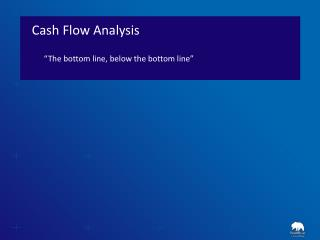DownloadDownload PresentationCash Flow Analysis

# Cash Flow Analysis

Download Presentation## Cash Flow Analysis

- - - - - - - - - - - - - - - - - - - - - - - - - - - E N D - - - - - - - - - - - - - - - - - - - - - - - - - - -
##### Presentation Transcript

1. Cash Flow Analysis “The bottom line, below the bottom line”

2. Cash Flow AnalysisPractical Use • Why are Cash Flows important? • Why bother?

3. Cash Flow AnalysisPractical Use • Close Look at CF fro OPS (in Millions) • What questions are raised?

4. Perfect Timing!

5. Cash Realization Ratio (CRR)Quality of Earnings • How to calculate: • CRR = (Cash from Ops) / (N.I.) • What does it tell? • Income independence from non-cash sources or non-repeatable sources (ex: mark-to-market accounting, gain on asset sale, etc…) • What a company wants? • CRR > 1 • For AmerBran: • \$574, 128 / \$328,773 = 1.7 (Great)

6. Coverage RatiosTimes Interest Earned (TIE) • How to calculate: • TIE = EBIT / Interest Payable • CF based TIE = CF from Ops / Interest Payables • What does it tell: • Ability to cover interest charges (bankruptcy) • Why use CF-Ops and not EBIT: Focus on cash (Ignore depreciation/Accounting write-offs) • What a company wants: • TIE >> 1 ( < 1 = Solvency issues)

7. Coverage RatiosTimes Interest Earned (TIE) • For AmerBran: • Estimation: • LTL + STD = \$1,311,450 • If 10% interest => Liability of \$131,145 • TIE = \$603,331 / \$ 131,145 = 4.6 • CF-TIE = \$574,128 / \$ 131,145 = 4.3

8. Coverage RatiosFix Charges Ratio (FCR) • Same principal as TIE Ratio • How to calculate: • FCR = EBIT / Fix Charges • CF based TIE = CF from Ops / Fix Charges • What does it tell? • Ability to cover fix charges • Low Fix Charges Ration could lead to: • breaches of contract penalties / Lawsuits • loose capabilities (eviction, lease repossessions) • Asset Deterioration (no \$ to repair) • Why use CF-Ops and not EBIT? • Focus on cash • What a company wants? • TIE >> 1 ( < 1 = Solvency issues)

9. Free Cash Flow (FCF) • How to calculate: • FCF = OPS CF – (KTLO + Debt Service + Dividends) • What does it tell? • Capacity to maintain (or increase) dividends • What a company wants? • Free Cash Flow > 0

10. Free Cash Flow (FCF) For AmerBran: • Assume Annual Depreciation is typical Asset Replacement: \$115,974 • Assume 10% interest on LT/ST Debt: \$131,145 • Disclosed Dividend: \$216,158 • FCF = \$574,128 – (\$115,974 + \$131,145 + \$216,158) = \$110,851 Conclusion: • Dividends seems sustainable

11. Sources & Uses of Cash

12. Sources of Cash • Conclusions on sources? • Borrowing comes with Liabilities • Stock issue dilutes ownership • Asset disposal impairs capabilities • Sales of investments in non-repeatable • Cash from Operations: • Fairly repeatable • No strings attached

13. Uses of Cash Cash Ratio: - \$28,912 / \$1,625,218 = 0.018 Quick Ratio: - \$785,064 / \$1,625,218 = 0.48 Questions Raised?

14. Conclusion • Facts on AmerBrand: • Quality Earnings (Cash Realization Ratio = 1.7) • Not exposed to imminent bankruptcy (TIE = 4.3) • Questionable cash management (QR = 0.48 yet only 1% cash preserved) • Sustainable Divided (FCF = \$110,851) • For Management: • Suggest revision of cash management/investment strategies • For Investors: • Solid operations and sustainable dividends • Buy as long as economy is doing well • Keep an eye on company’s cash levels